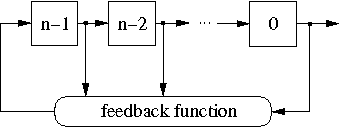# Maximum Period NLFSRs

Below we give a complete list of n-bit NLFSRs with the period 2n−1, n < 26, for the following types of feedback functions:
x0 ⊕ xa ⊕ xb ⊕ xc· xd
x0 ⊕ xa ⊕ xb· xc ⊕ xd· xe
x0 ⊕ xa ⊕ xb ⊕ xc ⊕ xd ⊕ xe· xf
x0 ⊕ xa ⊕ xb ⊕ xc · xd · xe
where "⊕" is an XOR (addition modulo 2) and " · " is an AND.

The maximum possible period for a n-bit NLFSR is 2n. The NLFSRs listed below do not include all-0 state in their longest cycle of states. They can be extended to have the period 2n by adding to their feedback function a product-term ¬x1 · ¬x2 · ... · ¬xn−1, where "¬" is a NOT. This increases the circuit complexity of feedback functions, which is undesirable for many applications. For this reason, we prefer to focus on NLFSRs with the period 2n−1.

The results given below were computed by a brute-force simulation which feasible for n < 26 only. FPGAs make possible finding larger maximum period NLFSRs, as shown in this article.

#### INTRODUCTION TO NLFSRs

The general structure of an n-bit NLFSR is shown in Figure 1. It consists of n binary storage elements, called bits or stages, and a feedback function f(x0,x1,...xn−1). For each i ∈ {0,1,...,n−1 }, the state variable xi represents the value of the bit i. At each clock cycle, the content of the register is shifted one bit right. The value of the bit n−1 is updated according to the feedback function f.Figure 1: The general structure of an n-bit NLFSR.

The configuration shown in Figure 1 is usually referred to as the Fibonacci configuration. One can also represent an NLFSR in the Galois configuration, in which any stage has its own feedback function.

Figure 2 shows an example of a 4-bit NLFSR with the feedback function f = x0 ⊕ x1 ⊕ x2 ⊕ x1· x2.Figure 2: An example of a 4-bit NLFSR

#### FORMAT OF FEEDBACK FUNCTIONS

In the format we use in the list below, the function f = x0 ⊕ x1 ⊕ x2 ⊕ x1· x2 from the example in Figure 2 is represented as 0,1,2,(1,2). Indexes of variables of each product-term are separated by a comma. If a product-term has of more than one variable, we put round brackets around the indexes of its variables.

#### BASIC AND IMPLIED CASES

The state transition graph of an n-bit NLFSR is branchless, i.e. consists of pure cycles, if and only if its feedback function is of type
(1) f = x0 ⊕ g(x1,x2,...,xn−1)
where the function g(x1,x2,...,xn−1) does not depend on the variable x0.

Each NLFSR N with the feedback function of type (1) has a revserse, a complement, and a reverse-complement NLFSRs which generate sequences which are reverse, complement and reverse-complement of sequences generated by N, respectively. In the list below, we show only the basic case. Other three cases are not included.

4-bit NLFSRs
5-bit NLFSRs
6-bit NLFSRs
7-bit NLFSRs
8-bit NLFSRs
9-bit NLFSRs
10-bit NLFSRs
11-bit NLFSRs
12-bit NLFSRs
13-bit NLFSRs
14-bit NLFSRs
15-bit NLFSRs
16-bit NLFSRs
17-bit NLFSRs
18-bit NLFSRs
19-bit NLFSRs
20-bit NLFSRs
21-bit NLFSRs
22-bit NLFSRs
23-bit NLFSRs
24-bit NLFSRs
25-bit NLFSRs

#### REFERENCE

E. Dubrova, "A List of Maximum Period NLFSRs", Cryptology ePrint Archive, Report 2012/166, March 2012, http://eprint.iacr.org/2012/204.

#### CONTACT PERSON

Elena Dubrova
School of Information and Communication Technology
Royal Institute of Technology (KTH)
Stockholm, Sweden
dubrova@kth.se